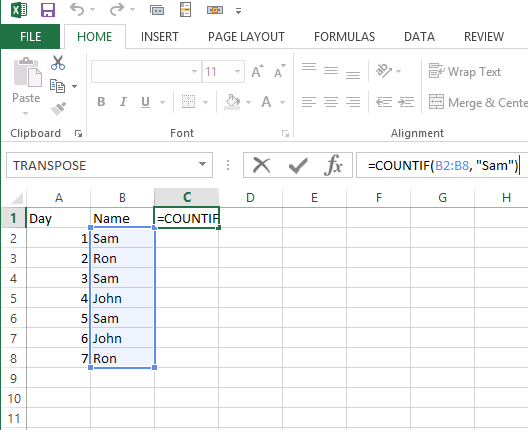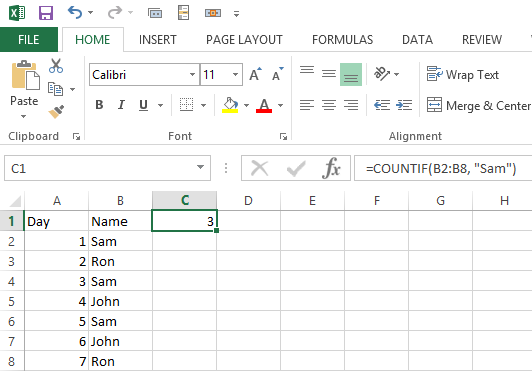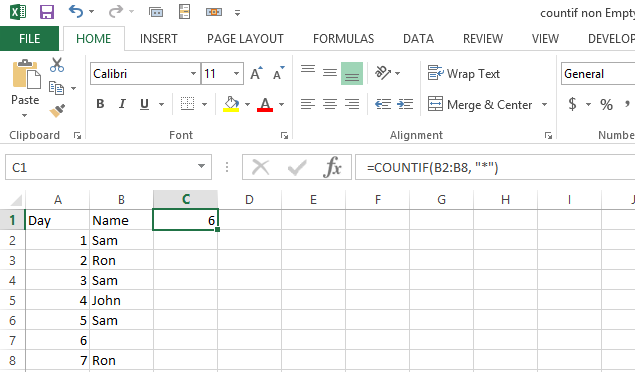### How To Count Non Blank Cells In Excel

In this article we will create the COUNTIF function in Excel for non empty cells. You will learn how to count non blank cells.

Before doing that I will explain the basics about the COUNTIF formula.

The Syntax of the COUNTIF formula is:

=COUNTIF(range, criteria)

So we have 2 arguments to be passed in this:

1. Range: It will be the range which we want to be checked for or say the range from which we will count the cells.
2. Criteria: It will be the condition which needs to be satisfied for counting the values from the range that we already specified in the range parameter.

Please find below a simple example for the same:In this example we have the 7 days duty chart for 3 persons.

We have to count the number of duties for a person say “Sam”

And we use the formula as below: =COUNTIF(B2:B8, “Sam”)

Here range is: B2:B8

Criteria is : “Sam”

So we the the result as: 3 (because Sam is repeated 3 times under the range)We can use the same formula to check any name starting with “Sa” like:

=COUNTIF(B2:B8, “Sa*”)

Now coming to the point we need to count for non blank cells. We can use: =COUNTIF(range,”*”)As we have only 6 entries, the result is 6 which is correct. But if we use numbers instead of blank it fails because number is treated like blank.Another formula is: =COUNTIF(range,”<>”)Now the result is good. Number has been counted to the result of formula. This is how to count non blank cells.

## Template

```Further reading: